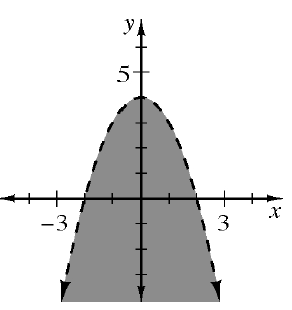### Home > CCG > Chapter Ch10 > Lesson 10.2.1 > Problem10-71

10-71.

A graph of an inequality is shown at right. Decide if each of the points $(x, y)$ listed below would make the inequality true or not. For each point, explain how you know.If a point makes the inequality true, it must be in the shaded region.

1. $(1, 1)$

True

1. $(−3, 2)$

False

1. $(−2, 0)$

False

1. $(0, −2)$

True